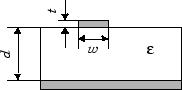# txlineMicrostrip

Create microstrip transmission line

## Description

Use the `txlineMicrostrip` object to create a microstrip transmission line. The cross-section of a microstrip transmission line is shown in this figure. The physical characteristics of the microstrip transmission line include the microstrip width (w), the microstrip thickness (t), the substrate height (d), and the relative permittivity constant (ε).## Creation

### Syntax

``txline = txlineMicrostrip``
``txline = txlineMicrostrip(Name,Value)``

### Description

````txline = txlineMicrostrip` creates a default microstrip transmission line object.```

example

````txline = txlineMicrostrip(Name,Value)` sets Properties using one or more name-value pairs. For example, ```txline = txlineMicrostrip('Width',0.0046)``` creates a microstrip transmission line with a width of `0.0046` meters.```

## Properties

expand all

Name of the microstrip transmission line, specified as a string scalar or a character vector.

Example: `'Name','microstrip1'`

Example: `txline.Name = 'microstrip1'`

Data Types: `char` | `string`

Physical length of the microstrip transmission line, specified as a positive scalar in meters.

Example: `'LineLength',0.0200`

Example: `txline.LineLength = 0.0200`

Data Types: `double`

Physical width of the microstrip transmission line, specified as a positive scalar in meters.

Example: `'Width',0.0008`

Example: `txline.Width = 0.0008`

Data Types: `double`

Physical height of the conductor or dielectric thickness, specified as a positive scalar in meters.

Example: `'Height',0.000835`

Example: `txline.Height = 0.000835`

Data Types: `double`

Physical thickness of the microstrip transmission line, specified as a positive scalar in meters.

Example: `'Thickness',0.000008`

Example: `txline Thickness = 0.000008`

Data Types: `double`

Relative permittivity of the dielectric, specified as a positive scalar.

Example: `'EpsilonR',8.8`

Example: `txline.EpsilonR = 8.8`

Data Types: `double`

Loss angle tangent of the dielectric, specified as a nonegative scalar.

Example: `'LossTangent',1`

Example: `txline.LossTangent = 1`

Data Types: `double`

Conductivity of the conductor, specified as a nonnegative scalar in Siemens per meter (S/m).

Example: `'SigmaCond',2`

Example: `txline.SigmaCond = 2`

Data Types: `double`

Stub transmission line termination, specified as `'NotApplicable'`, `'Open'`, or `'Short'`.

Example: `'Termination','Short'`

Example: `txline.Termination = 'Short'`

Data Types: `char`

Type of stub, specified as `'NotAStub'`, `'Series'`, or `'Shunt'`.

Example: `'StubMode','Series'`

Example: `txline.StubMode = 'Series'`

Data Types: `char`

Number of input and output ports, returned as a positive scalar.

Data Types: `double`

Terminals of the microstrip transmission line, returned as a cell array of strings.

Data Types: `char` | `string`

## Object Functions

 `sparameters` S-parameter object `groupdelay` Group delay of S-parameter object or RF filter object or RF Toolbox circuit object `noisefigure` Calculate noise figure of transmission lines `getZ0` Calculate characteristic impedance of transmission line object `circuit` Circuit object `clone` Create copy of existing circuit element or circuit object

## Examples

collapse all

Create a microstrip transmission line using these specifications:

• Width - 0.08 mm

• Height - 1.6 mm

• Line length - 12.2777 mm

• Thickness - 10 mm

• Conductivity - 5.88e7 S/m

• EpsilonR - 3.9 F/m

```microstriptxline = txlineMicrostrip('Width',0.08e-3,'Height',1.6e-3,... 'LineLength',12.2777e-3,'Thickness',10e-6,'EpsilonR',3.9,'SigmaCond',5.88e7)```
```microstriptxline = txlineMicrostrip: Microstrip element Name: 'Microstrip' Width: 8.0000e-05 Height: 0.0016 Thickness: 1.0000e-05 EpsilonR: 3.9000 LossTangent: 0 SigmaCond: 58800000 LineLength: 0.0123 Termination: 'NotApplicable' StubMode: 'NotAStub' NumPorts: 2 Terminals: {'p1+' 'p2+' 'p1-' 'p2-'} ```

Calculate the s-parameters of the transmission line at 10 GHz.

`sparam = sparameters(microstriptxline,10e9,50);`

Calculate the group delay of the transmission line at 10 GHz.

`gd = groupdelay(microstriptxline,10e9,'Impedance',50)`
```gd = 4.2440e-11 ```